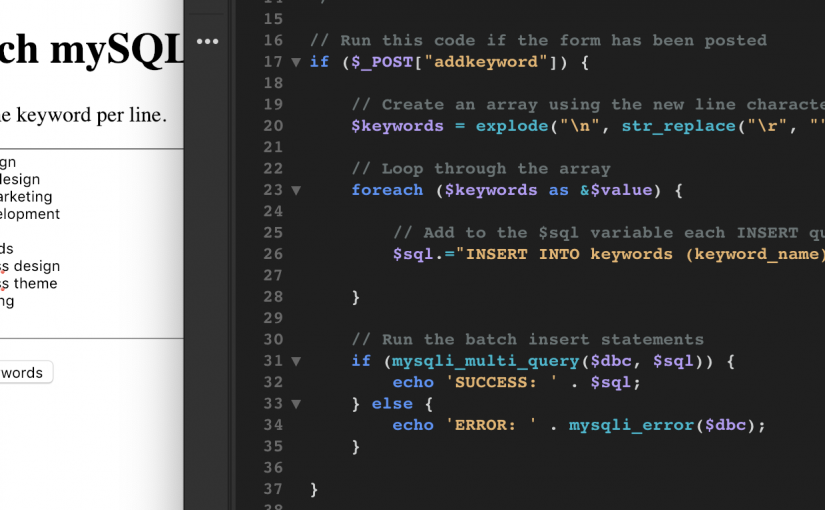# Adding Batch / Multiple SQL Insert Queries with PHP’s mysqli_multi_query()

I had a project which required to insert a batch of mySQL database rows in one go with PHP.

I firstly wrote the script to simply loop through an array and then make a new call to the database for each row, but then I stopped and thought that might become an issue performance wise with so many hits on the database. So after a little research I discovered the mysqli_multi_query() option.

With mysqli_multi_query() you can have a whole bunch of INSERT statements together and then run them with a single submission to mySQL.

In the below example, we first create the \$sql variable and keep adding as many additional INSERT statements as we like. Then we simply run it all.

```// Add to the \$sql variable with each additional INSERT statement
\$sql="INSERT INTO table (name) VALUES ('name1')";
\$sql.="INSERT INTO table (name) VALUES ('name2')";
\$sql.="INSERT INTO table (name) VALUES ('name3')";

// Run the batch insert statements
if (mysqli_multi_query(\$dbc, \$sql)) {
echo 'SUCCESS: ' . \$sql;
} else {
echo 'ERROR: ' . mysqli_error(\$dbc);
}```

Now, the above is a very basic example which could be repurposed for a number of different options.

## A Batch Keyword Textarea Example

To take the concept a little further, here is an example of a batch keyword adding form, which would take each line of a textarea and add these as individual table rows.

Like a shortcut? For a couple of bucks you can download the full working script here

Firstly, you will need to setup a sample database table in mySQL to have somewhere to add the records to. Here is some SQL you can run.

```// SQL to create the table in your database
CREATE TABLE `keywords` (
`id` int(11) NOT NULL,
`keyword_name` varchar(100) NOT NULL
) ENGINE=MyISAM DEFAULT CHARSET=latin1;```

Next, let’s add a real basic HTML form, just with a textarea and a button.

```<form method="POST" action="" name="batchkeywordadd">
<p><textarea name="keywords" value="" rows="10" ></textarea></p>
</form>```

The above form will just POST the form values back to the same page, so now we would need to add some PHP functionality in order to do something with this data.

```// Run this code if the form has been posted

// Create an array using the new line characters
\$keywords = explode("\n", str_replace("\r", "", \$_POST['keywords']));

// Loop through the array
foreach (\$keywords as &\$value) {

// Add to the \$sql variable each INSERT query
\$sql.="INSERT INTO keywords (keyword_name) VALUES ('" . mysqli_real_escape_string(\$dbc, \$value) . "'); ";

}

// Run the batch insert statements
if (mysqli_multi_query(\$dbc, \$sql)) {
echo 'SUCCESS: ' . \$sql;
} else {
echo 'ERROR: ' . mysqli_error(\$dbc);
}

}```

So, in the above we are taking the contents of the textarea and using the explode function to make this into an array. Having this as an array then allows us to loop through each keyword using a foreach loop, building our INSERT query and adding to the \$sql variable with each loop through.

Again, if you would like to purchase the full working script click here

I hope this helps someone out 🙂